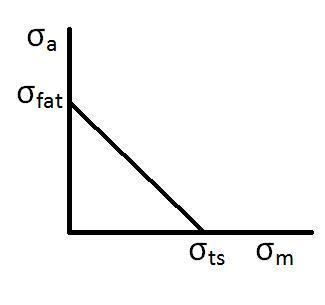# Goodman relation

Updated on
Covid-19In materials science and fatigue, the Goodman relation is an equation used to quantify the interaction of mean and alternating stresses on the fatigue life of a material.

A Goodman diagram, sometimes called a Haigh diagram or a Haigh-Soderberg diagram, is a graph of (linear) mean stress vs. (linear) alternating stress, showing when the material fails at some given number of cycles.

A scatterplot of experimental data shown on such a plot can often be approximated by a parabola known as the Gerber line, which can in turn be (conservatively) approximated by a straight line called the Goodman line.

## Mathematical description

The Goodman relation can be represented mathematically as:

σ a = σ fat × ( 1 σ m σ ts ) .

Where σ a is the alternating stress, σ m is the mean stress, σ fat is the fatigue limit for completely reversed loading, and σ ts is the ultimate tensile strength of the material. The general trend given by the Goodman relation is one of decreasing fatigue life with increasing mean stress for a given level of alternating stress. The relation can be plotted to determine the safe cyclic loading of a part; if the coordinate given by the mean stress and the alternating stress lies under the curve given by the relation, then the part will survive. If the coordinate is above the curve, then the part will fail for the given stress parameters.

## References

Similar Topics
Selvam (2005 film)
João Paulo Bravo
Valeri Simeonov
Topics